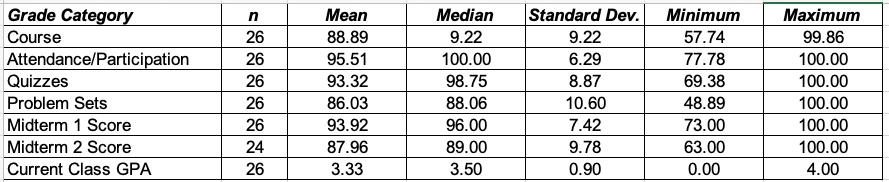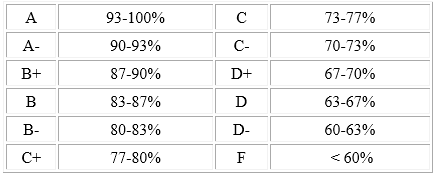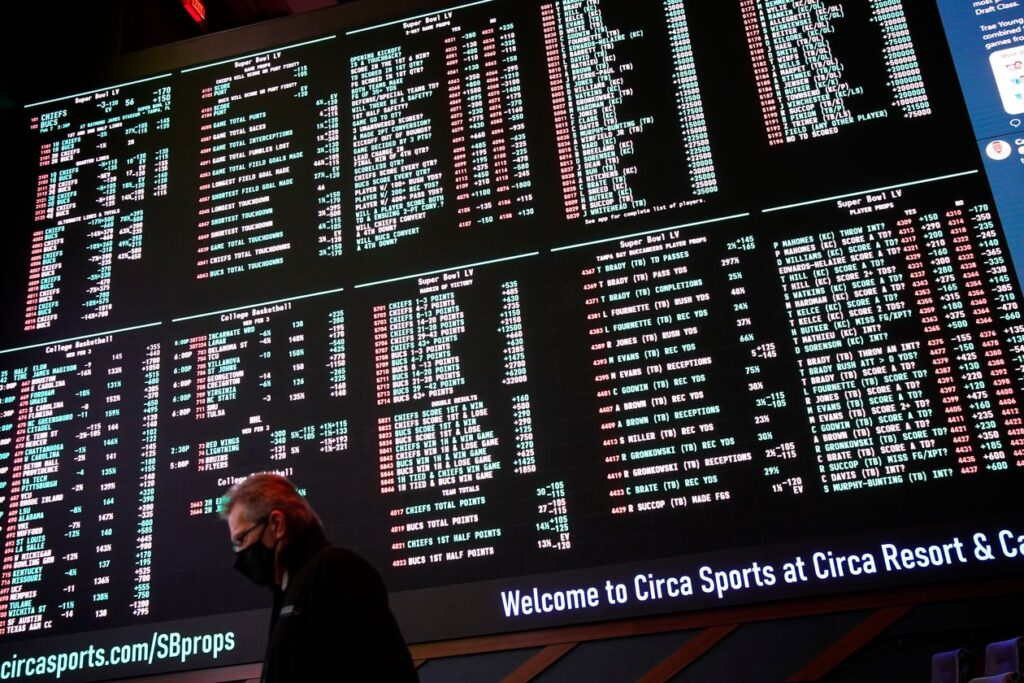## Midterm 2 and Current Course Grades in Finance 4366

I just uploaded the Midterm 2 Exam grades, along with attendance, quiz, problem set, and current Finance 4366 course grades to Canvas.

As indicated in the course syllabus, final numeric course grades will be determined according to the following equation:

Final Course Numeric Grade =.10(Attendance and Participation) +.10(Quizzes) +.20(Problem Sets) + Max{.20(Midterm Exam 1) +.20(Midterm Exam 2) +.20(Final Exam),.20(Midterm Exam 1) +.40(Final Exam),.20(Midterm Exam 2) +.40(Final Exam)}

As I noted in my February 1st blog posting entitled “Finance 4366 Grades on Canvas”, as the spring semester progresses and I continue to collect grades in the attendance, quiz, problem set, and exam categories, then the course grade listed on Canvas will dynamically incorporate that information on a timely basis for each student; now that we have Midterm 2 Exam grades, the equation that I am currently using (until the Final Exam) is as follows:

Course Numeric Grade after Midterm 2 = (.10(Attendance and Participation) +.10(Quizzes) +.20(Problem Sets) +.20(Midterm 1+.20(Midterm 2))/.8

There are 26 students enrolled in Finance 4366; here are the current grade statistics:From this table, you can get a good idea concerning how your grades compare with all other students who are currently enrolled in Finance 4366. You can also see where you stand in terms of a hypothetical letter grade for Finance 4366 by comparing your course grade with the letter grade schedule which appears in the course syllabus:If you are disappointed by your performance to date in Finance 4366, keep in mind that the final exam grade automatically double counts in place of a lower midterm exam grade. In case if both midterm exam grades are lower than the final exam grade, then the final exam grade replaces the lower of the two midterm exam grades.

If any of you would like to have a chat with me about your grades, by all means, then set up a Zoom appointment with me.

## Extra Credit Opportunity: Free Enterprise Forum with Glenn Loury, “Capitalism and Race”I am pleased to announce the Baugh Center’s upcoming Free Enterprise Forum with Glenn Loury, Merton P. Stoltz Professor of the Social Sciences at Brown University. The lecture, “Capitalism and Race,” is Thursday, April 22, at 5:30pm CST via Zoom. The event is co-sponsored by Baylor’s Department of Economics.

Professor Loury is one of the world’s leading experts on the economic and social aspects of race. He will discuss recent developments in race relations including the concept of antiracism, the shift of emphasis within organizations and institutions from diversity to equity and inclusion, and the role of markets in addressing racial bias.

Professor Loury has been on the faculty of Brown University since 2005. He was previously at Boston University where he served as University Professor and Founder and Director of the Institute on Race and Social Division. He has also taught at Harvard, Northwestern, and Michigan. He received his PhD in Economics from MIT. He has published widely in the areas of applied microeconomic theory, game theory, industrial organization, natural resource economics, and the economics of race and inequality. He is a Fellow of the American Academy of Arts and Sciences and the Econometric Society and also served as Vice President of the American Economic Association, and is a recipient of the Guggenheim Fellowship.

As a prominent social critic and public intellectual, writing mainly on the themes of racial inequality and social policy, Professor Loury has published over 200 essays and reviews in journals of public affairs in the U.S. and abroad. He is a member of the Council on Foreign Relations, is a contributing editor at The Boston Review, and was for many years a contributing editor at The New Republic. Professor Loury’s books include One by One, From the Inside Out: Essays and Reviews on Race and Responsibility in America (The Free Press, 1995 – winner of the American Book Award and the Christianity Today Book Award); The Anatomy of Racial Inequality (Harvard University Press, 2002); Ethnicity, Social Mobility and Public Policy: Comparing the US and the UK (ed., Cambridge University Press, 2005); and, Race, Incarceration and American Values (MIT Press, 2008).

Since this panel discussion has the makings of an extra-credit opportunity for Finance 4366, let’s have it! You can earn extra credit by attending this Zoom webinar and reporting on what you learn. If you decide to take advantage of this extra-credit opportunity, I will use the grade you earn on your report to replace your lowest quiz grade in Finance 4366 (assuming that your grade on the extra credit is higher than your lowest quiz grade). Submit your report as a (PDF formatted) 1-2 page executive summary. In order to receive credit, the report must be uploaded to the Assignments section of the Course Canvas page by no later than 5 pm CST on Monday, April 26 (Click on the Assignment entitled “Free Enterprise Forum with Glenn Loury, ‘Capitalism and Race’, April 22, 2021“).

Registration is required at https://bit.ly/FEF_Loury. After registering, you will receive a link to the Zoom webinar.

## Today’s spreadsheet modeling of risk neutral vs. true probabilities

My blog posting entitled “Actual versus True Probability that an option expires in the money” provides the necessary details for calculating risk neutral and true probabilities related to lognormally distributed asset prices and options which are based on such assets.  Here’s a screenshot of the spreadsheet that we worked on today:In this spreadsheet, the Asset Price (S), Exercise Price (K),$\mu$,$\sigma$, r, the annual mean log return (${\mu - .5{\sigma ^2}}$), and T are the problem parameters.  So we are studying a European call option which is currently in-the-money, and wish to determine its price, as well as the risk neutral and true probabilities associated with expiring in-the-money.  I also provide the price of an otherwise identical put option, and quite trivially, the probabilities (risk neutral and true) of the put expiring in-the-money are just equal to 1 minus the corresponding call option probabilities.

The${d_1}$ and${d_2}$ values work out to  .6664 and .4164, respectively.  Using the normsdist function in Excel, these${d_1}$ and${d_2}$ values imply an option delta of .7474, and a risk neutral probability of expiring in-the-money of .6615. The true probability of expiring in-the-money is obtained by calculating$N\left( {\displaystyle\frac{{\ln \left( {S/K} \right) + \left( {\mu - .5{\sigma ^2}} \right)T}}{{\sigma \sqrt T }}} \right)$, returning .7569.

## Risk Neutral versus True (Actual) Probability that an option expires in the money

Both the Cox-Ross-Rubinstein (discrete-time) and Black-Scholes-Merton (continuous-time) option pricing equations are based on risk neutral as opposed to “true” probabilities. The primary difference between the CRR and BSM equations is that CRR is based on binomial risk neutral probabilities, whereas BSM is based on normal risk neutral probabilities.  However, in the limit, CRR and BSM prices converge since CRR binomial probabilities converge into BSM normal probabilities.

Keeping this in mind, consider the BSM equation for the price of a call option:$C = SN\left( {{d_1}} \right) - K{e^{ - rT}}N({d_2}),$

where${d_1} = \displaystyle\frac{{\ln \left( {S/K} \right) + \left( {r + .5{\sigma ^2}} \right)T}}{{\sigma \sqrt T }},$ and${d_2} = {d_1} - \sigma \sqrt T = \displaystyle\frac{{\ln \left( {S/K} \right) + rT}}{{\sigma \sqrt T }}.$

In this equation,$N\left( {{d_1}} \right)$ corresponds to the option’s “delta”, whereas$N\left( {{d_2}} \right)$ corresponds to the risk neutral probability that the option will expire in-the-money.

In order to determine the “true” probability of expiring in-the-money, all one has to do is replace the riskless rate of interest “r”  in the expression for$d_2$ with the continuously compounded expected return on the underlying asset for the option, which is${\mu - .5{\sigma ^2}}$.  Keep in mind that the riskless rate of interest “r” corresponds to the expected return on the underlying asset in a risk neutral economy, whereas${\mu - .5{\sigma ^2}}$ corresponds to the continuously compounded expected return on the underlying asset in a risk averse economy.  Thus, the “true” probability that the call option will expire in the money is given by$N\left( {\displaystyle\frac{{\ln \left( {S/K} \right) + \left( {\mu - .5{\sigma ^2}} \right)T}}{{\sigma \sqrt T }}} \right)$.

## You Can Bet on the Vaccine, Literally

This WSJ  article tells a compelling story about how prediction markets offer important tools for managing risk.  For example, consider the following quote from this article:

…investors can bet on a contract “Will 100 million people have received a dose of an approved COVID-19 vaccine in the US by April 1, 2021?” (At this writing, the betting suggests a likelihood slightly above 1 in 3.)